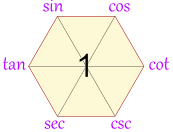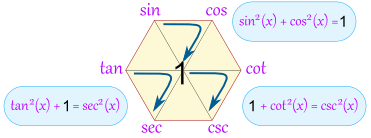# Basics of Trigonometry

Check out this site for nice simple and clear descriptions of different aspects of Trigonometry.    http://www.mathsisfun.com/algebra/trigonometry-index.html

including the piece below on the Magic Hexagon.

# Magic Hexagon for Trig Identities

 This hexagon is a special diagram to help you remember many trigonometric identities## Building It: The Quotient Identities

 Start with:tan(x) = sin(x) / cos(x)(This one you just have to remember)Then add: cot (which is cotangent) opposite tan csc (which is cosecant) next, and sec (which is secant) lastTo help you remember: the “co” functions are all on the right

OK, we have now built our hexagon, what do we get out of it?

Well, we can now follow “around the clock” (either direction) to get all the “Quotient Identities”:

 Clockwise Anticlockwise tan(x) = sin(x) / cos(x) sin(x) = cos(x) / cot(x) cos(x) = cot(x) / csc(x) cot(x) = csc(x) / sec(x) csc(x) = sec(x) / tan(x) sec(x) = tan(x) / sin(x) cos(x) = sin(x) / tan(x) sin(x) = tan(x) / sec(x) tan(x) = sec(x) / csc(x) sec(x) = csc(x) / cot(x) csc(x) = cot(x) / cos(x) cot(x) = cos(x) / sin(x)

## Product Identities

The hexagon also shows that a function between any two functions is equal to them multiplied together (if they are opposite each other, then the “1” is between them):Example: tan(x)cos(x) = sin(x) Example: tan(x)cot(x) = 1

Some more examples:

• sin(x)csc(x) = 1
• tan(x)csc(x) = sec(x)
• sin(x)sec(x) = tan(x)

## But Wait, There is More!

You can also get the “Reciprocal Identities”, by going “through the 1”Here you can see that sin(x) = 1 / csc(x)

Here is the full set:

• sin(x) = 1 / csc(x)
• cos(x) = 1 / sec(x)
• cot(x) = 1 / tan(x)
• csc(x) = 1 / sin(x)
• sec(x) = 1 / cos(x)
• tan(x) = 1 / cot(x)

## Bonus!

AND we also get these:Examples: sin(30°) = cos(60°) tan(80°) = cot(10°) sec(40°) = csc(50°)

## Double Bonus: The Pythagorean Identities

The Unit Circle shows us that

sin2 x + cos2 x = 1

The magic hexagon can help us remember that, too, by going around any of these three triangles:And we have: sin2(x) + cos2(x) = 1 1 + cot2(x) = csc2(x) tan2(x) + 1 = sec2(x)

You can also travel backwards around a triangle. For example:

• 1 – cos2(x) = sin2(x)

Trigonometry Tutorial from Engineers Ireland. Lasts an hour and a quarter but you can always look at it in smaller bits at a time.

From http://www.skoool.ie

Trigonometry is one of the most important topics in Higher Level Leaving Cert maths. It is also one of the most problematic for many students. It is hard to avoid trigonometry, as well as accounting for two full questions out of seven in Section A of Paper 2, trigonometry is required in many other areas of the course, e.g., complex numbers, differentiation, integration and vectors.

Originally trigonometry was purely the study of triangles, but it has been expanded greatly since then. Our course reflects this development. We study triangles, sectors, circles, trigonometry ratios as well as the more general trigonometry functions. We learn how to prove trigonometry identities and how to solve trigonometry equations. We also examine inverse trigonometry functions and investigate a well-known trigonometry limit.

The study of Senior Cycle (Leaving Cert) Trigonometry can be divided into the following sections.

1. Fundamentals of Trigonometry2. Trigonometry of the triangle and other figures3. Trigonometric Identities4. Trigonometric Equations5. Inverse Trigonometric Functions and Limits